Gaussian Processes¶

Introduction¶

Gaussian Processes have been used in supervised, unsupervised, and even reinforcement learning problems and are described by an elegant mathematical theory (for an overview of the subject see [1, 4]). They are also very attractive conceptually, since they offer an intuitive way to define priors over functions. And finally, since Gaussian Processes are formulated in a Bayesian setting, they come equipped with a powerful notion of uncertainty.

Happily, Pyro offers some support for Gaussian Processes in the pyro.contrib.gp module. The goal of this tutorial is to give a brief introduction to Gaussian Processes (GPs) in the context of this module. We will mostly be focusing on how to use the GP interface in Pyro and refer the reader to the references for more details about GPs in general.

The model we’re interested in is defined by

$f \sim \mathcal{GP}\left(0, \mathbf{K}_f(x, x')\right)$

and

$y = f(x) + \epsilon,\quad \epsilon \sim \mathcal{N}\left(0, \beta^{-1}\mathbf{I}\right).$

Here $$x, x' \in\mathbf{X}$$ are points in the input space and $$y\in\mathbf{Y}$$ is a point in the output space. $$f$$ is a draw from the GP prior specified by the kernel $$\mathbf{K}_f$$ and represents a function from $$\mathbf{X}$$ to $$\mathbf{Y}$$. Finally, $$\epsilon$$ represents Gaussian observation noise.

We will use the radial basis function kernel (RBF kernel) as the kernel of our GP:

$k(x,x') = \sigma^2 \exp\left(-\frac{\|x-x'\|^2}{2l^2}\right).$

Here $$\sigma^2$$ and $$l$$ are parameters that specify the kernel; specifically, $$\sigma^2$$ is a variance or amplitude squared and $$l$$ is a lengthscale. We’ll get some intuition for these parameters below.

Imports¶

First, we import necessary modules.

In :

from __future__ import print_function
import os
import matplotlib.pyplot as plt
import torch

import pyro
import pyro.contrib.gp as gp
import pyro.distributions as dist

smoke_test = ('CI' in os.environ)  # ignore; used to check code integrity in the Pyro repo
assert pyro.__version__.startswith('0.3.1')
pyro.enable_validation(True)       # can help with debugging
pyro.set_rng_seed(0)


Throughout the tutorial we’ll want to visualize GPs. So we define a helper function for plotting:

In :

# note that this helper function does three different things:
# (i) plots the observed data;
# (ii) plots the predictions from the learned GP after conditioning on data;
# (iii) plots samples from the GP prior (with no conditioning on observed data)

def plot(plot_observed_data=False, plot_predictions=False, n_prior_samples=0,
model=None, kernel=None, n_test=500):

plt.figure(figsize=(12, 6))
if plot_observed_data:
plt.plot(X.numpy(), y.numpy(), 'kx')
if plot_predictions:
Xtest = torch.linspace(-0.5, 5.5, n_test)  # test inputs
# compute predictive mean and variance
if type(model) == gp.models.VariationalSparseGP:
mean, cov = model(Xtest, full_cov=True)
else:
mean, cov = model(Xtest, full_cov=True, noiseless=False)
sd = cov.diag().sqrt()  # standard deviation at each input point x
plt.plot(Xtest.numpy(), mean.numpy(), 'r', lw=2)  # plot the mean
plt.fill_between(Xtest.numpy(),  # plot the two-sigma uncertainty about the mean
(mean - 2.0 * sd).numpy(),
(mean + 2.0 * sd).numpy(),
color='C0', alpha=0.3)
if n_prior_samples > 0:  # plot samples from the GP prior
Xtest = torch.linspace(-0.5, 5.5, n_test)  # test inputs
noise = (model.noise if type(model) != gp.models.VariationalSparseGP
else model.likelihood.variance)
cov = kernel.forward(Xtest) + noise.expand(n_test).diag()
samples = dist.MultivariateNormal(torch.zeros(n_test), covariance_matrix=cov)\
.sample(sample_shape=(n_prior_samples,))
plt.plot(Xtest.numpy(), samples.numpy().T, lw=2, alpha=0.4)

plt.xlim(-0.5, 5.5)


Data¶

The data consist of $$20$$ points sampled from

$y = 0.5\sin(3x) + \epsilon, \quad \epsilon \sim \mathcal{N}(0, 0.2).$

with $$x$$ sampled uniformly from the interval $$[0, 5]$$.

In :

N = 20
X = dist.Uniform(0.0, 5.0).sample(sample_shape=(N,))
y = 0.5 * torch.sin(3*X) + dist.Normal(0.0, 0.2).sample(sample_shape=(N,))

plot(plot_observed_data=True)  # let's plot the observed data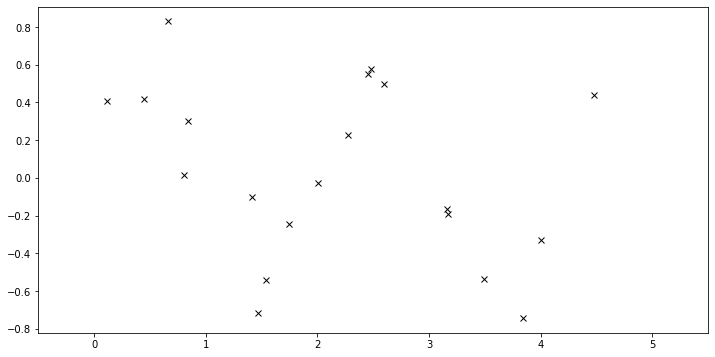Define model¶

First we define a RBF kernel, specifying the values of the two hyperparameters variance and lengthscale. Then we construct a GPRegression object. Here we feed in another hyperparameter, noise, that corresponds to $$\epsilon$$ above.

In :

kernel = gp.kernels.RBF(input_dim=1, variance=torch.tensor(5.),
lengthscale=torch.tensor(10.))
gpr = gp.models.GPRegression(X, y, kernel, noise=torch.tensor(1.))


Let’s see what samples from this GP function prior look like. Note that this is before we’ve conditioned on the data. The shape these functions take—their smoothness, their vertical scale, etc.—is controlled by the GP kernel.

In :

plot(model=gpr, kernel=kernel, n_prior_samples=2)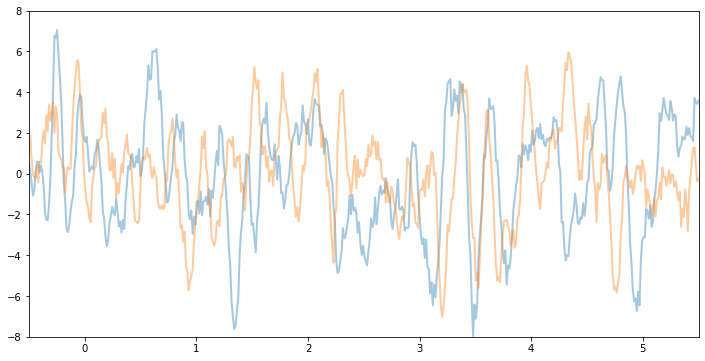For example, if we make variance and noise smaller we will see function samples with smaller vertical amplitude:

In :

kernel2 = gp.kernels.RBF(input_dim=1, variance=torch.tensor(0.1),
lengthscale=torch.tensor(10.))
gpr2 = gp.models.GPRegression(X, y, kernel2, noise=torch.tensor(0.1))
plot(model=gpr2, kernel=kernel2, n_prior_samples=2)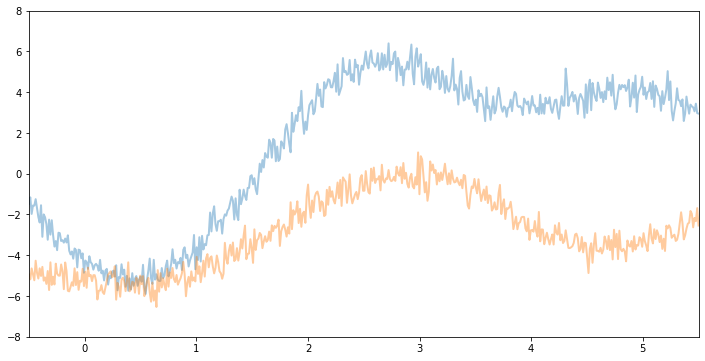Inference¶

In the above we set the kernel hyperparameters by hand. If we want to learn the hyperparameters from the data, we need to do inference. In the simplest (conjugate) case we do gradient ascent on the log marginal likelihood. In pyro.contrib.gp, we can use any PyTorch optimizer to optimize parameters of a model. In addition, we need a loss function which takes inputs are the pair model and guide and returns an ELBO loss (see SVI Part I tutorial).

In :

optimizer = torch.optim.Adam(gpr.parameters(), lr=0.005)
loss_fn = pyro.infer.Trace_ELBO().differentiable_loss
losses = []
num_steps = 2500 if not smoke_test else 2
for i in range(num_steps):
loss = loss_fn(gpr.model, gpr.guide)
loss.backward()
optimizer.step()
losses.append(loss.item())

In :

# let's plot the loss curve after 2500 steps of training
plt.plot(losses);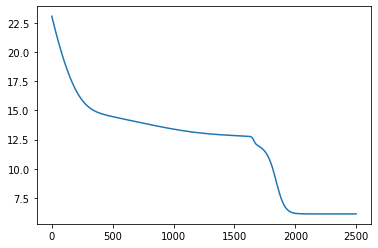Let’s see if we’re learned anything reasonable:

In :

plot(model=gpr, plot_observed_data=True, plot_predictions=True)Here the thick red curve is the mean prediction and the blue band represents the 2-sigma uncertainty around the mean. It seems we learned reasonable kernel hyperparameters, as both the mean and uncertainty give a reasonable fit to the data. (Note that learning could have easily gone wrong if we e.g. chose too large of a learning rate or chose bad initital hyperparameters.)

Note that the kernel is only well-defined if variance and lengthscale are positive. Under the hood Pyro is using PyTorch constraints (see docs) to ensure that hyperparameters are constrained to the appropriate domains. Let’s see the constrained values we’ve learned.

In :

gpr.kernel.variance.item()

Out:

0.20291312038898468

In :

gpr.kernel.lengthscale.item()

Out:

0.5022153854370117

In :

gpr.noise.item()

Out:

0.04272938147187233


The period of the sinusoid that generated the data is $$T = 2\pi/3 \approx 2.09$$ so learning a lengthscale that’s approximiately equal to a quarter period makes sense.

Fit the model using MAP¶

We need to define priors for the hyperparameters.

In :

# Define the same model as before.
kernel = gp.kernels.RBF(input_dim=1, variance=torch.tensor(5.),
lengthscale=torch.tensor(10.))
gpr = gp.models.GPRegression(X, y, kernel, noise=torch.tensor(1.))

# note that our priors have support on the positive reals
gpr.kernel.set_prior("lengthscale", dist.LogNormal(0.0, 1.0))
gpr.kernel.set_prior("variance", dist.LogNormal(0.0, 1.0))

loss_fn = pyro.infer.Trace_ELBO().differentiable_loss
losses = []
num_steps = 2500 if not smoke_test else 2
for i in range(num_steps):
loss = loss_fn(gpr.model, gpr.guide)
loss.backward()
optimizer.step()
losses.append(loss.item())
plt.plot(losses);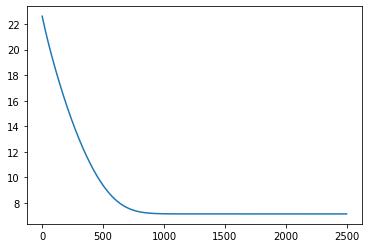In :

plot(model=gpr, plot_observed_data=True, plot_predictions=True)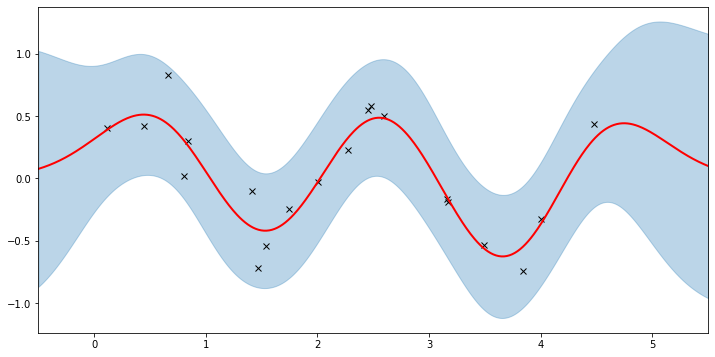Let’s inspect the hyperparameters we’ve learned:

In :

print('variance = {}'.format(gpr.kernel.variance))
print('lengthscale = {}'.format(gpr.kernel.lengthscale))
print('noise = {}'.format(gpr.noise))

variance = 0.2447132021188736
lengthscale = 0.5217674970626831
noise = 0.04222296178340912


Note that the MAP values are different from the MLE values due to the prior.

Sparse GPs¶

For large datasets computing the log marginal likelihood is costly due to the expensive matrix operations involved (e.g. see Section 2.2 of ). A variety of so-called ‘sparse’ variational methods have been developed to make GPs viable for larger datasets. This is a big area of research and we won’t be going into all the details. Instead we quickly show how we can use SparseGPRegression in pyro.contrib.gp to make use of these methods.

First, we generate more data.

In :

N = 1000
X = dist.Uniform(0.0, 5.0).sample(sample_shape=(N,))
y = 0.5 * torch.sin(3*X) + dist.Normal(0.0, 0.2).sample(sample_shape=(N,))
plot(plot_observed_data=True)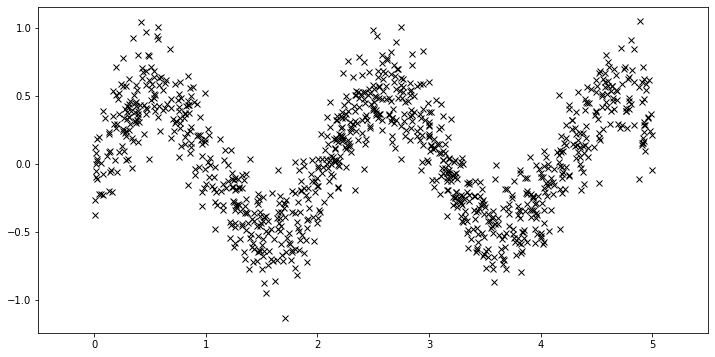Using the sparse GP is very similar to using the basic GP used above. We just need to add an extra parameter $$X_u$$ (the inducing points).

In :

# initialize the inducing inputs
Xu = torch.arange(20.) / 4.0

# initialize the kernel and model
kernel = gp.kernels.RBF(input_dim=1)
# we increase the jitter for better numerical stability
sgpr = gp.models.SparseGPRegression(X, y, kernel, Xu=Xu, jitter=1.0e-5)

# the way we setup inference is similar to above
loss_fn = pyro.infer.Trace_ELBO().differentiable_loss
losses = []
num_steps = 2500 if not smoke_test else 2
for i in range(num_steps):
loss = loss_fn(sgpr.model, sgpr.guide)
loss.backward()
optimizer.step()
losses.append(loss.item())
plt.plot(losses);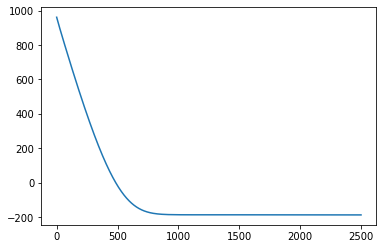In :

# let's look at the inducing points we've learned
print("inducing points:\n{}".format(sgpr.Xu.data.numpy()))
# and plot the predictions from the sparse GP
plot(model=sgpr, plot_observed_data=True, plot_predictions=True)

inducing points:
[0.03058701 0.25927886 0.48168573 0.76160955 1.0478696  1.3311042
1.6020889  1.8753066  2.0877776  2.342296   2.5872447  2.8880212
3.1459439  3.3801115  3.6570277  3.9431984  4.1874566  4.47123
4.721643   4.96045   ]We can see that the model learns a reasonable fit to the data. There are three different sparse approximations that are currently implemented in Pyro:

• “DTC” (Deterministic Training Conditional)
• “FITC” (Fully Independent Training Conditional)
• “VFE” (Variational Free Energy)

By default, SparseGPRegression will use “VFE” as the inference method. We can use other methods by passing a different approx flag to SparseGPRegression.

More Sparse GPs¶

Both GPRegression and SparseGPRegression above are limited to Gaussian likelihoods. We can use other likelihoods with GPs—for example, we can use the Bernoulli likelihood for classification problems—but the inference problem becomes more difficult. In this section, we show how to use the VariationalSparseGP module, which can handle non-Gaussian likelihoods. So we can compare to what we’ve done above, we’re still going to use a Gaussian likelihood. The point is that the inference that’s being done under the hood can support other likelihoods.

In :

# initialize the inducing inputs
Xu = torch.arange(10.) / 2.0

# initialize the kernel, likelihood, and model
kernel = gp.kernels.RBF(input_dim=1)
likelihood = gp.likelihoods.Gaussian()
# turn on "whiten" flag for more stable optimization
vsgp = gp.models.VariationalSparseGP(X, y, kernel, Xu=Xu, likelihood=likelihood, whiten=True)

# instead of defining our own training loop, we will
# use the built-in support provided by the GP module
num_steps = 1500 if not smoke_test else 2
losses = gp.util.train(vsgp, num_steps=num_steps)
plt.plot(losses);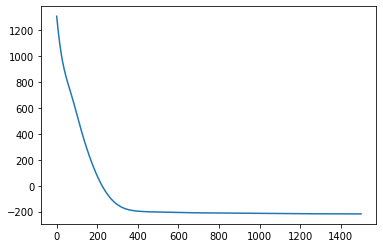In :

plot(model=vsgp, plot_observed_data=True, plot_predictions=True)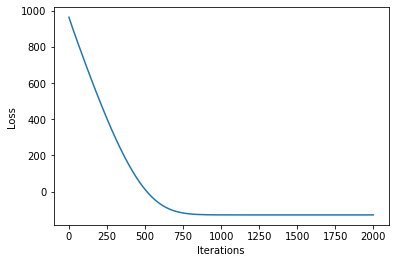That’s all there is to it. For more details on the pyro.contrib.gp module see the docs. And for example code that uses a GP for classification see here.

Reference¶

 Deep Gaussian processes and variational propagation of uncertainty, Andreas Damianou

 A unifying framework for sparse Gaussian process approximation using power expectation propagation, Thang D. Bui, Josiah Yan, and Richard E. Turner

 Scalable variational Gaussian process classification,     James Hensman, Alexander G. de G. Matthews, and Zoubin Ghahramani

 Gaussian Processes for Machine Learning,     Carl E. Rasmussen, and Christopher K. I. Williams

 A Unifying View of Sparse Approximate Gaussian Process Regression, Joaquin Quinonero-Candela, and Carl E. Rasmussen